Start typing, then use the up and down arrows to select an option from the list.# GOB Chemistry

Learn the toughest concepts covered in your GOB - General, Organic, and Biological Chemistry class with step-by-step video tutorials and practice problems.

Acids and Bases

A Buffer is a solution composed of a weak acid with its conjugate base.

1

#### concept

Buffers Concept 13m
Play a video:

Buffers resist drastic changes to the pH if a strong acid or strong base is added.If a strong base is added then the buffer resists a pH change by having the weak acid neutralize it.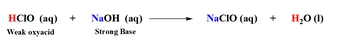If a strong acid is added then the buffer resists a pH change by having the conjugate base neutralize it.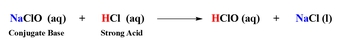2
Problem

Which one of the following combinations does not create a buffer?

3
Problem

Which of the following combinations can result in the formation of a buffer?

As we stated earlier a buffer is composed of a weak acid and its conjugate base, but there are actually three ways to create a buffer.

4

#### concept

Buffers Concept 24m
Play a video:
Hey, guys, In this new video, we're gonna take a look at what are the different ways to create a buffer? All right, we know what a buffer is. A buffer is made up of a weak acid and conjugate base or a conjugate acid and a weak base. So that's what a buffer is. But there are three ways to make a buffer. It's gonna be important that you guys recognize these three ways. Now we're gonna say the first way is obvious. Ah, buffer is made up of a weak acid and it's consequent base. So one way to make a buffer is just mixing those two things together. So we're gonna say, mixing a weak acid and its conjugate base. So, for example, what we need to realize here is that we're gonna say in this case, Ah, good buffer, an ideal buffer. So ideal buffer means that this is the best type of buffer. An ideal buffer is when our weak acid equals r conjugate base there the same amount. So let's say we have 0.1 Mueller HF and 0.1 Moeller and A F, and we should realize that the numbers could be different for them, but they work best when they're the same. We're gonna say this has to do with our buffer range. What you should realize here, a good buffer has to fall within the range of 10 to or 1 to 10. Okay. So what do I mean by that? I mean this. So 10 toe one that means are weak. Acid at most could only be 10 times more than my conjugate base. If it falls without that outside that range, it'll be a bad buffer. Okay, so good buffers between 10 toe attend to one ratio or 1 to 10 ratio. And the 1 to 10 now, the weak acid is one, and the conjugate base is 10 times that. Okay, So, again, this is the buffer range. Ah, good. Buffer falls within this range. If it falls outside that range, it'll still be a buffer. It'll just be a very bad buffer. And remember, an ideal buffer is the best buffer. That's when both are the same. Now, connected to this also is another topic which is called our buffer capacity. Now we're gonna say buffer capacity. All it means is the more concentrated my weak acid and conjugate base, the better my buffer. And this makes sense because remember, what is a buffer doing? It's trying to neutralize the strong acid in the strong base that you add to them, and they can't do their job effectively if there's a little bit off them. So to fight such strong acids and strong bases, you need a lot of weak acid and a lot of conjugate base. The more the better, the more they can better defend themselves. So here we're gonna say, this is good because they're both the same number. But this is better because it's more concentrated. So this has a better buffer capacity. Oops. Okay, so buffer capacity, the more concentrated the better. Now, if we go back to this ideal buffer, we're gonna say that's when they both equal each other. We're gonna say that this is found at our half equivalents point when we reached the half equivalents point. That's when we have an ideal buffer. We'll talk more detail on that when we get to titrate Asian graphs. When we get to the Thai tray shin graphs of buffers, we'll take a close look and see what do I mean by the half equivalents point. And why is this an ideal buffer? So just for right now, write a little note on that and ideal buffers when they're both equal. This happens at the half equivalents point. So remember the difference between buffer capacity and buffer range, uh, the best buffers when they're both equal in amount. The buffer rain says there could be a 10 to 1 ratio. Once it's outside that 10 to 1 ratio. The buffer is still a buffer, but it's gonna be a bad buffer. It'll get destroyed very quickly by a strong acid or base that we add.

The first and most obvious way to create a buffer is to simply combine a weak acid and its conjugate base. In this case, a buffer is most ideal when both components are highly concentrated and equal to one another.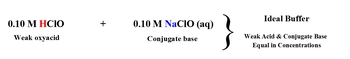The weak acid and conjugate base can be different from one another by up to a magnitude of 10. This is called the buffer range. If they are different by more than 10 then this is considered a bad buffer.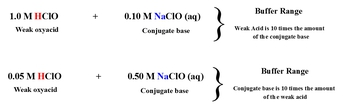5

#### concept

Buffers Concept 31m
Play a video:
Now, the second way to make a buffer is to mix some strong acid with some weak base. But here's the thing about this type we're gonna say here the week base has to be higher in amount. Must be hiring amount. If the weak base is equal to the strong acid, it will destroy the buffer. If the strong acid is greater than my weak base, it will destroy the buffer. Okay, so the week base has to always be higher than the strong acid. Otherwise, the buffer will be destroyed. So if we take a look at an example, we can say Here we have one mole of HF and 1.5 moles off NH three and actuaries are weak base and there's more of it. Our units here could be moles or mole Arat E. In this case, this would be a buffer if we have one mole of HF and one more of NH three no longer a buffer because the strong species is equal to my week Species, if I have one point Not sorry, this is HCL. Actually H f is a weak acid. So disregard that hcl if I have one more 1.5 moles of HCL and still one more off and H three. This would also no longer be a buffer. Your strong species cannot be equal to or greater than your week species. If it is, your buffer gets destroyed. So remember that the week species has to be higher in amount in the strong species.

The second method in creating a buffer is mixing a strong acid with a weak base. In this case since we have a strong species mixing with a weak species then we must make sure the weak species is higher in amount.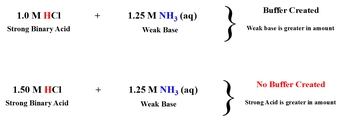6

#### concept

Buffers Concept 41m
Play a video:
And then finally the last type. We just said strong acid in week base. So let's reverse it. Weak acid and strong base. So example here we could have one point. Let's do different. Number 250 Mueller HF. Now that's a weak acid and 1.0 Mueller N a O h. Okay, so now are weak. Acid is definitely larger, an amount so again here we need the week species higher in amount. So this would be a buffer. Got to remember that. So if we go back, first way is to mix weak acid and contacted base here. They could be equal to or differ from each other. But the good buffers are within the range of 10 to 1 in the next to the week. Species has to be higher than the strong species. Otherwise, it won't be a buffer. You have to remember all three cases because you'll be tested on them. And if you don't know which is which, you won't be able to get the correct answer. So just remember we cast the contact base that could be equal. It could be different asl, long as it's within the range of 10 to 1. It's a good buffer. If it's outside that range, it's a bad buffer. Case two and three the week Species have to be higher than the strong species. The strong species cannot be equal to or greater. If it is, the buffer gets destroyed. Remember these principles and the help guide you to see if you have a buffer or not.

The third method in creating a buffer is mixing a strong base with a weak acid. In this case since we have a strong species mixing with a weak species then we must make sure the weak species is higher in amount.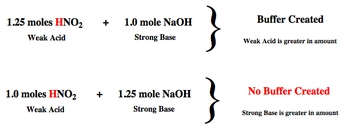7

#### example

Buffers Example 13m
Play a video:
Hey, guys, In this new video, we're gonna put to practice some of the principles we learned in creating a buffer. So, for example, when it says which of the following combinations can result in the formation of a buffer, so we should realize here is in every example, we have 0.1 moles off Hypo Cloris acid now hypochlorite acid, it says acid. So we know it's some type of acid. It has oxygen, so it's an oxy acid. Remember, if we do the math one oxygen minus one hydrogen gives us zero left over, so hypochlorite sass. It is a weak acid, so every single one of these is a weak acid. And because of that, you have to remember what two ways can we make a buffer if we have a weak acid? So remember the two ways is if we have a weak acid and its conjugate base, and the second ways if we have a weak acid and a strong base, But in this second case, the week species has to be higher in amount. So let's take a look at the what's mixing with here. We have a wage which is a strong base. Here we have HCL, which is a strong binary acid. Here in H three is a neutral amine. So it's a week base and anyway, which again is a strong base. So we're looking for the weak acid to be paired up with the conjugate base or the strong base so automatically these tour out because we can't pair up a weak acid with a strong acid or weak acid with a weak base has to be a conjugal base or strong base. Now, in the first one, we actually have more moles of the strong species, so this would not be a buffer. This would actually destroy the buffer. But for the last one, we have mawr of the week species than the strong species, so it would be the last one that makes a buffer. So again, remember the three principles that we learned in creating a buffer apply them to this type of question. Now, for practice one, I want you guys to figure out which one of these combinations will result in a buffer here. Remember, the word off means multiply. So if you multiply these two numbers together, you can help to find moles because remember, moles equals leaders times molar ity. So divide each of these by 1000 multiplied by the molar iti to have moles. You could also keep it in milliliters and multiply times the polarity. But then that would give you Millie moles. So I don't know if you guys want to deal with Millie moles. It's basically the same thing, but you don't have to do it that way, So divide them by 1000. The MLS to get leaders multiply times similarity to give you moles. Remember the three combinations that could result in the formation of a buffer. So check for each one of these. Look to see. Do you have a weak acid and conjugate base? Do you have a weak acid and strong base, or do you have a strong acid and weak base? Look for these combinations. If you don't see them, don't bother doing the math. So I would recommend looking at each compound first and seeing if they match up with any of these combinations. If they do look more deeply look to see doesn't help to create a buffer. If it doesn't match one of these three combinations, don't even bother looking at it. Don't do the math at all. And remember one key thing. I'll give you guys a hint. You have to find out to make sure that the you have the correct concentration of your strong bases. What did we say about strong basis? The concentration I give you is dependent on how maney ions you have, of which particular four ions doing that will help toe get the correct answer. Once you're done with this, come back and click on the explanation button and watch me explain how toe best approach this problem. Good luck, guys.
8
Problem

Which of the following combinations can result in the formation of a buffer?

9
Problem

A buffer solution is comprised of 50.0 mL of a 0.100 M HC2H3O2 and 60.0 mL of a 0.100 M NaC2H3O2. Which of the following actions would completely destroy the buffer?